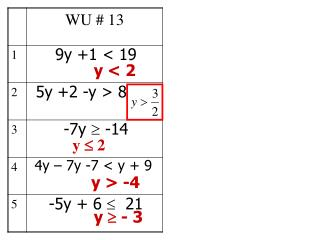Download Presentation9y +1 < 199y +1 < 19 - PowerPoint PPT Presentation

Download Presentation9y +1 < 19
An Image/Link below is provided (as is) to download presentation

Download Policy: Content on the Website is provided to you AS IS for your information and personal use and may not be sold / licensed / shared on other websites without getting consent from its author. While downloading, if for some reason you are not able to download a presentation, the publisher may have deleted the file from their server.

- - - - - - - - - - - - - - - - - - - - - - - - - - - E N D - - - - - - - - - - - - - - - - - - - - - - - - - - -
Presentation Transcript

1. y < 2 y  2 y > -4 y  - 3 9y +1 < 19 5y +2 -y > 8 -7y  -14 4y – 7y -7 < y + 9 -5y + 6  21

2. 4.5 Using Inequalities • Goal: To translate phrases to mathematical inequalities and then solve

3. The small 2 letter word IS…. Is huge! It tells you it is either =, >, < , ≥, or≤ If there is not an “is” then it is strictly an operation (+, -,X, or ÷)

4. Note card

5. 6 -6 -4 -2 0 2 4 “x” is 2 x = 2 “x” is at least 2 x  2

6. 6 -6 -4 -2 0 2 4 “x” is 2 x = 2 “x” is at least 2 “x” is at most 2 x  2 x  2

7. A number “y”is less than 4 y < 4 A number “y” is 3 less than 4 y = 4 - 3

8. 12 more than twice a number is less than 20 12+ 2n < 20 < 20

9. The sum of three consecutive integers is less than 75. What are the greatest possible values of these integers? Let x = the first consecutive integer x + (x + 1) + (x + 2) < 75 3x + 3 < 75 X x < 24 23, 24, 25 24, 25, 26

10. The sum of three consecutive integers is less than 59. What are the greatest possible values of these integers? Let x = the first consecutive integer x + (x + 1) + (x + 2) < 59 3x + 3 < 59 3x < 56 x < 18.67 18, 19, 20

11. 2. Find the greatest possible pair of integers such that one integer is 3 more than twice the other and their sum is less than 42. Let x = the “other” integer the “first” integer is 3 + 2x x + (3 + 2x) < 42 3x + 3 < 42 X x < 13 12 27 13,29 ?

12. The length of a rectangle is 5 cm more than twice the width, and the perimeter is greater than 28 cm. What is the width of the rectangle? Let w = the width length is 5 + 2w 2w + 2(5 + 2w) > 28 6w + 10 > 28 w > 3

13. The base of a triangle is 8 cm. What height will make the area greater than 32 cm2? Let h = the height Area = ½ • b • h  ½ • 8 • h 4h > 32 h > 8

14. Gail works for a vending company. She gets paid \$64 per week plus 20% of her total sales. How much will her total sales for the week have to be in order for Gail to make at least \$200? Let s = total sales Pay = 64 + 0.20(s) 64 + 0.2s  200 0.2s  136 5 • • 5 s  680

15. How long must the sides of an equilateral triangle be in order for the perimeter to be greater than 45 m? Let s = each side 3s > 45 s > 15

16. Assignment:Page 189(2-26) evenWrite the questions for 2-14 and just write the data for 16-26